# KSEEB Solutions for Class 9 Science Chapter 3 Atoms and Molecules

In this chapter, we provide KSEEB SSLC Class 9 Science Chapter 3 Atoms and Molecules for English medium students, Which will very helpful for every student in their exams. Students can download the latest KSEEB SSLC Class 9 Science Chapter 3 Atoms and Molecules pdf, free KSEEB SSLC Class 9 Science Chapter 3 Atoms and Molecules pdf download. Now you will get step by step solution to each question.

### KSEEB Solutions for Class 9 Science Chapter 3 Intext Questions

KSEEB Solutions For Class 9 Science Chapter 3 Question 1.
In a reaction, 5.3 g of sodium carbonate reacted with 6 g of ethanoic acid. The products were 2.2 g of carbon dioxide, 0.9 g water and 8.2 g of sodium ethanoate. Show that these observations are in agreement with the law of conservation of mass.
Sodium carbonate + ethanoic acid ➝ sodium ethanoate + carbon dioxide + water
Sodium carbonate + ethanoic acid ➝ sodium ethanoate + carbon dioxide + water
5.3 + 6 ➝ 8.2 + 2.2 + 0.9
= 11.3 g = 11.3 g
Weight of reactants is equal to weight of products. This observation is in agreement with the law of conservation of mass.

KSEEB Solutions For Class 9 Science Atoms And Molecules Question 2.
Hydrogen and Oxygen combine in the ratio or 1 : 8 by mass to form water. What mass of oxygen gas would be required to react completely with 3 g of hydrogen gas?
24 g of oxygen gas would be required to react completely with 3 g of hydrogen gas. Because in water the ratio of the mass of hydrogen to the mass of oxygen is always 1 : 8.

Atoms And Molecules Class 9 Notes KSEEB Question 3.
Which postulate of Dalton’s atomic theory is the result of the law of conservation of mass?
The relative number and kinds of atoms are constant in a given compound. This postulate is the result of the law of conservation of mass.

KSEEB Solutions For Class 9 Science Question 4.
Which postulate of Dalton’s atomic theory can explain the law of definite proportions?
Atoms combine in the ratio of small whole numbers to form compounds. This postulate explains the law of definite proportions.

Atoms And Molecules Class 9 KSEEB Solutions Question 1.
Define the atomic mass unit.
One atomic mass unit is a mass unit equal to exactly one-twelfth (1/12th) the mass of one atom of carbon-12.

KSEEB Solutions For Class 9 Science Structure Of Atom Question 2.
Why is it not possible to see an atom with naked eyes?
Atoms are very small, they are smaller than anything that we can imagine or compare with. Therefore it is not possible to see an atom with naked eyes.

KSEEB Solutions Class 9 Science Question 1.
Write down the formulae of:

1. sodium oxide
2. alluminium chloride
3. sodium sulphide
4. magnesium hydroxide

1. sodium oxide: Na2O
2. aluminium chloride: Al2Cl3
3. sodium sulphide : NaS
4. magnesium hydroxide: Mg(OH)2

KSEEB Solutions Science Class 9 Question 2.
Write down the names of compounds represented by the following formulae:

1. Al2(SO4)3
2. CaCl2
3. K2SO4
4. KNO3
5. CaCO3

1. Al2(SO4)3: Aluminium sulphate
2. CaCl2: Calcium chloride
3. K2SO4: potassium sulphate
4. KNO3: potassium nitrate
5. CaCO3: calcium carbonate

KSEEB Solutions For Class 9 Question 3.
what is meant by the term chemical formula?
The chemical formula of a compound is a symbolic representation of its composition.

KSEEB Solutions For Class 9 Science Chapter 3 Atoms And Molecules Question 4.
How many atoms are present in a
i) H2S molecule and
ii) PO43- ion?
i) H2S molecule: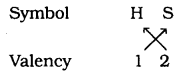ii) PO43- ion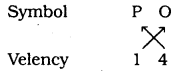Calculate the molecular masses of
H2, O2, Cl2, CO2, CH4, C2H6, C2H4, NH3, CH3OH.
i) H2
= 2 × H
= 2 × 1
= 2u

ii) O2
= 2 × O
= 2 × 16
= 32u

iii) Cl2
= 2 × Cl
= 2 × 35.5
= 7lu

iv) CO2
= 1 × 12 + 2 × O
= 1 × 12 + 2 × 16
= 12 + 32
= 44u

v) CH4
= 1 × 12 + 4 × 1
= 12 + 4
= 16u

vi) C2H6
= 2 × 12 + 6 × 1
= 24 + 6
= 30u

vii) C2H4
= 2 × 12 + 4 × 1
= 24 + 4
= 28u

viii) NH3
= 14 × 1 + 3 × 1
= 14 + 3
= 17u

ix) CH3OH
= 12 × 1 + 3 × 1 + 16 × 1 + 1 × 1
= 12 + 3 + 16 + 1
= 32u

KSEEB Solutions For 9th Class Science Question 2.
Calculate the formula unit masses of ZnO, Na2O, K2CO3 given atomic masses of Zn = 65u, Na = 23u, K = 39u, C = 12u and O = 16u.
i) ZnO
(Automic mass of Zn) + (Automic mass of O)
= 65 + 16 = 81u.

ii) Na2O
(2 × Automic mass of Na) + (Automic mass of O)
= 2 × 23 + 1 × 16
= 46 + 16
= 62u.

iii) K2CO3
(2 × Atomic mass of K)+ (Atomic mass of C) + (3 × Atomic mass of oxygen)
= 2 × 39 + 12 × 1 + 16 × 3
= 78 + 12 + 48
= 138u.

Karnataka State Syllabus For Class 9 Science Question 1.
If one mole of carbon atoms weighs 12 gms, what is the mass (in gms) of 1 atom of carbon?
Number of moles = n
Given mass = m
molar mass = M
Given the number of particles = N
Avogadro number of particles = N0
i) Atomic mass of carbon = 12u.
Atomic mass of one mole of carbon=12g
But Atomic mass of carbon = 12 g mass of 6.022 × 1023 carbon atoms = 12g.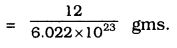∴ Mass of carbon atoms
= 1.9926 x 10-23 gm.

KSEEB Science Solutions For Class 9 Question 2.
Which has more number of atoms, 100 g, 100 gms of sodium or 100 gms of sodium or 100 gms of iron (given, the atomic mass of Na=23u, Fe = 56u).
Atomic mass of sodium = 23u (data)
It means the gram atomic mass of sodium = 23 gm
Now atoms present in 23 gm sodium = 6.022 × 1023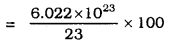It means Number of atoms in 100 gm sodium = 1.6753 × 1024
∴ 100 gm sodium has more number of atoms rather than 100 gms of iron.

### KSEEB Solutions for Class 9 Science Chapter 3 Textbook Exercises

9th Science KSEEB Solutions Question 1.
A 0.24 g sample of a compound of oxygen and boron was found by analysis to contain 0.096g of boron and 0.144 g of oxygen. Calculate the percentage composition of the compound by weight.
Mass of Boron = 0.096 g (Data)
Mass of Oxygen = 0.144g (Data)
Given mass = 0.24 g (Data)
∴ The percentage composition of Boron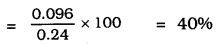∴ The percentage of O2 = 0.1440.24 × 100
= 60%.

KSEEB Solutions For Class 9th Science Question 2.
When 3.00 g of carbon is burnt in 8.009 of oxygen, 11.00 g of carbon dioxide is produced. What mass of carbon dioxide will be formed when 3.00 g of carbon is burnt in 50,000 g of oxygen? Which law of Chemical combination will govern your answer?
3.0 g of carbon is burnt in 8.00 g of oxygen, 11.00 g of carbon dioxide is produced.
But when 3 g of carbon is burnt in 50 g of oxygen, only 3 g of carbon reacts with 8 g of oxygen.
Remaining 42 gm of oxygen will not react.
11 gm of carbon dioxide is produced.
∴ Our answer obeys law of constant proportion.

KSEEB Solutions 9th Science Question 3.
What are polyatomic ions? Give examples.
A group of atoms carrying a charge is known as a polyatomic ion.

KSEEB Solutions For 9th Science Question 4.
Write the chemical formulae of the following.
(a) Magnesium chloride
(b) Calcium oxide
(c) Copper nitrate
(d) Aluminium chloride
(e) Calcium carbonate
(a) Magnesium chloride : MgCl2
(b) Calcium oxide : CaO
(c) Copper nitrate : Cu(NO3)2
(d) Aluminium chloride : AlCl3
(e) Calcium carbonate : CaCO3.

Give the names of the elements present in the following compounds.
(a) Quick lime
(b) Hydrogen bromide
(c) Baking powder
(d) Potassium sulphate
(a) Quick lime: Calcium, carbon, oxygen
(b) Hydrogen bromide: Hydrogen, Bromine
(c) Baking powder: Sodium, Bicarbonate
(d) Potassium sulphate: Potassium, Sulphur, Oxygen.

KSEEB 9th Science Solutions Question 6.
Calculate the molar mass of the following substances.
(a) Ethyne, C2H2
(b) Sulphur molecule, S8
(c) Phosphorus Molecule, P4 (Atomic mass of phosphorus = 31)
(d) Hydrochloric acid, HCl
(e) Nitric acid HNO3.
(a) Ethyne C2H2
Molar Mass = 2 × 12 + 2 × 1
= 24 + 2
= 26 g.

(b) Molar mass of Sulphur molecule
= 8 × 32
= 256 g.

(c) Molar Mass of Phosphorus molecule = 4 × 31
(Atomic mass of Phosphorus) = 124 g.

(d) Molar mass of Hydrochloric acid = HCl
= 1 + 35.5
= 36.5 g.

(e) Molar mass of HNO3
= 1 + 14 + 3 × 16
= 15 + 48
= 63 gm.

Class 9 Science ಉತ್ತರಗಳು Karnataka State Board Solutions Question 7.
What is the mass of –
(a) 1 mole of nitrogen atoms?
(b) 4 moles of aluminium atoms (Atomic mass of Aluminium = 27)
(c) 10 moles of Sodium Sulphite (Na2SO3)
(a) Mass of 1 mole of nitrogen= 14g.

(b) Mass of 4 moles of Aluminium
= 4 × 27
= 108 g.

(c) Mass of 10 moles of Sodium sulphite
= 10 × [2 × 23 + 32 + 3 × 16]
= 10 × 126
= 1260 gm.

9th Standard Science Notes Question 8.
Convert into mole:
(a) 12 g of oxygen gas
(b) 20 g of water
(c) 22 g of carbon dioxide
(a) 32gm of oxygen means 1 mole
12gm oxygen means 1232 mole
∴ 12g of oxygen gas = 0.375 mole
(b) 18 gm of water means = 1 mole
20 gm of water means 2018 mole = 1.11 mole
(c) 22 g of carbon dioxide means
2244 = 0.5 mole.

9th Standard Science Question Answer Question 9.
What is the mass of:
(a) 0.2 mole of oxygen atoms?
(b) 0.5 mole of water molecules?
(a) Mass of 1 mole of oxygen = 16 g
Mass of 0.2 mole of oxygen = 0.2 × 16
= 3.2 g.
(b) Mass of 1 molecule of water= 18 gm.
Mass of 0.5 mole of water = 0.5 × 18
= 9 gm.

Karnataka State Syllabus For Class 9 Science Notes Question 10.
Calculate the number of molecules of sulphur (S8) present in 16 g of solid sulphur.
One mole of Sulphur (S) = 8 × 32
= 256 gm.
256 g of solid sulphur = 6.022 × 1023 molecules.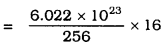= 3.76 × 1022
(Approximate)

Question 11.
Calculate the number of aluminium ions present in 0.0519 of aluminium oxide.
(Hint: The mass of an ion is the same as that of an atom of the same element. Atomic mass of Al = 27 u).
One mole of Aluminium oxide
= 2 × 27+ 3 × 16
= 54 + 48
= 102 g.
102 g of Al2O3 = 6.022 × 1023 moles (aluminium oxide)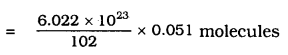It means Aluminium present in 0.051 gm
= 3.011 × 1020 Aluminium oxide molecules
Number of Al ions in one mole of Al2O3 = 2
∴ Number of Al Ions In 3.011 × 1026 molecules
0.051 Al2O3
= 2 × 3.011 × Number of Al ions In 3.011 × 1020
= 6.022 × 1020.

### KSEEB Solutions for Class 9 Science Chapter 3 Additional Questions

Question 1.
Write the symbols of the following:
a) Iron
c) Zinc
d) Oxygen
e) Chlorine
a) Iron = Fe
c) Zinc = Zn
d) Oxygen = O
e) Chlorine = Cl

Question 2.
What is a molecule?
The smallest particle of an element or a compound that is capable of independent existence and shows all the properties of that substance.

Question 3.
What is an ion?
An ion is a charged species present in metals and non-metals.

Question 4.
What is molecular mass?
The molecular mass of a substance is the sum of the atomic masses of all the atoms in a molecule of the substance.

Question 5.
What is a mole?
One mole of any species (atoms, molecules, ions, or particles) is that quantity in number having a mass equal to its atomic or molecular mass in grams.

### KSEEB Class 9 Science Atoms and Molecules Additional Questions and Answers

Question 1.
An element Z forms an oxide with formula Z2O3. What is its valency?
Valency is 3+

Question 2.
Mention the elements present in (1) quick line (2) sodium hydrogen carbonate.,
(1) Quick line (calcium oxide) (CaO) element present are calcium and oxygen.
(2) Sodium hydrogen carbonate (NaHCO3) elements are sodium, hydrogen, hydrogen, carbon and oxygen.

Question 3.
Calculate the total number of ions in 0.585 g of sodium chloride.
Gram formula mass of NaCl = 23 + 35-5 = 58.5 g
58.5 g of NaCl have ions = 2 × NA
58.5 g of NaCl have ions
=2×NA×0.58558⋅5=0⋅02×NA
= 0.02 × 6.022 x 1023
= 1.20 × 1022 ions

All Chapter KSEEB Solutions For Class 9 Science

—————————————————————————–

All Subject KSEEB Solutions For Class 9

*************************************************

I think you got complete solutions for this chapter. If You have any queries regarding this chapter, please comment on the below section our subject teacher will answer you. We tried our best to give complete solutions so you got good marks in your exam.

If these solutions have helped you, you can also share kseebsolutionsfor.com to your friends.

Best of Luck!!